Select Page

Part VI, Section a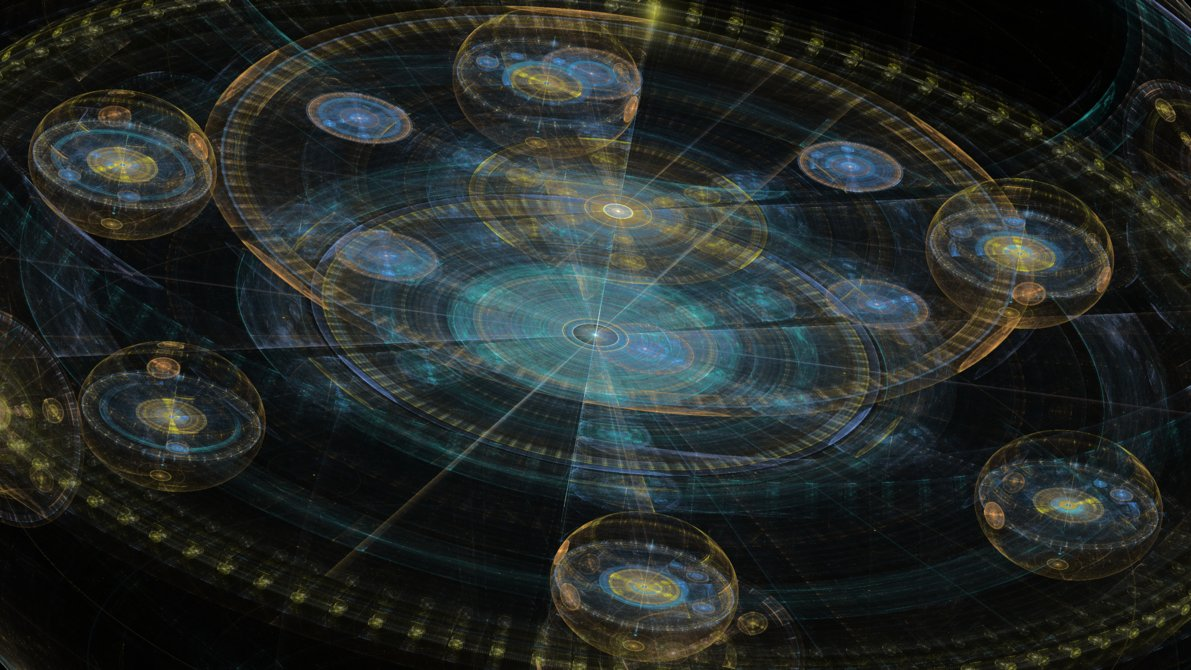Since the Torah has highlighted and documented this set of specific numbers, lets see if there is a mathematical correlation between them and the cosmos beyond the quantitative borders of the Torah. Once again, Number Theory provides evidence that the choice of these particular numbers might be proof of a coherent intentional and intelligent design. This proof would illustrate deep knowledge of the mathematical underpinnings of the universe and show us how to connect to it.
Besides utilizing the constants what could an advanced intelligence program into the Torah prove such asserted deep knowledge?
The answer is found in Bell Numbers.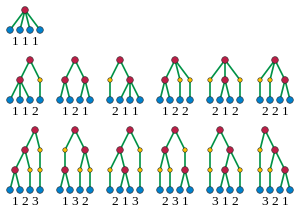Bell Numbers have to do with partition theory. They represent the number of ways a set of elements can be partitioned (divided or split) into non-empty sets. This connection makes perfect sense as we began our discussion with the partitioning of the 22 letters into two sets of 11 elements each. Then later we revealed some deeply hidden clues by partitioning the first 11 letters of the Torah into core syllables while translating them from base 3. In a sense, the Torah is one long 304,805-letter Name of G-d partitioned into words, verses, chapters, paragraphs, portions, all with purpose and meaning.
As we will see soon, all the key Torah elements can be split in two in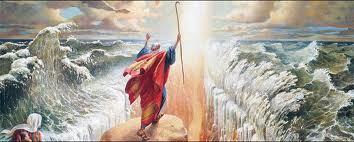very specific ways to reveal important information. This includes the 304,805 letters of the Torah, which can be partitioned into two sets: one of 300,000 elements, and the other with the 4,805 letter Pe’s (פ) that recur in the Torah. Think about it: Not only was the Torah arranged so that it would work out to a precise 58 elements, but also that 21 of its letters would add up to exactly 300,000 with the letter Shin (ש) of value 300 being the 21st Hebrew letter.
The connection to the 4,805 letter Pe’s (פ) in the 304,805 letters is obvious. The connection to the letter Shin (ש) of value 300 or the remaining 300,000 letters is a strong one.  So why? Is there something conveyed to us through this connection to the letter Shin (ש) of value 300 and it being the 21st Hebrew letter?
There are several Names of G-d contained in the Torah, yet of the 22 letters, the letter Shin (ש) is the only one that can be said to represent one. And not one, but four Names of G-d:
The numerical value 21 is that of the higher—higher as in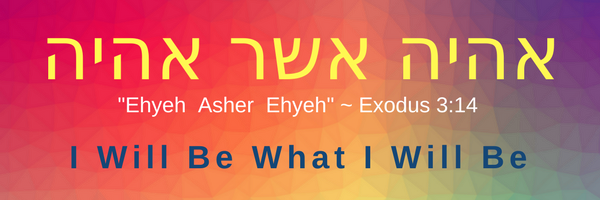dimensionality–Name of G-d, used only 7 times in the Torah, Ehyeh (אהיה);
There are several well established gematria ciphers dating back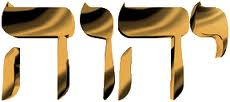thousands of years. After the standard one we outlined at the start of this paper, the next most commonly used one is gematria atbash, in which the value of the letters are the exact opposite of the standard values with the letter Alef(א) worth 1 transforms into the letter Tav (ת) and now worth 400. Using gematria atbash, the Tetragrammaton (יהוה) transforms into another form of the Tetragrammaton(מצפצ) of numerical value 300.  By the way, using gematria atbash the Name Ehyeh (אהיה) of value 21 becomes the Name (תצמצ) of numerical value 620, that of Keter, the highest level.
The letter Shin (ש) also represents the Name Shaddai (שדי) which is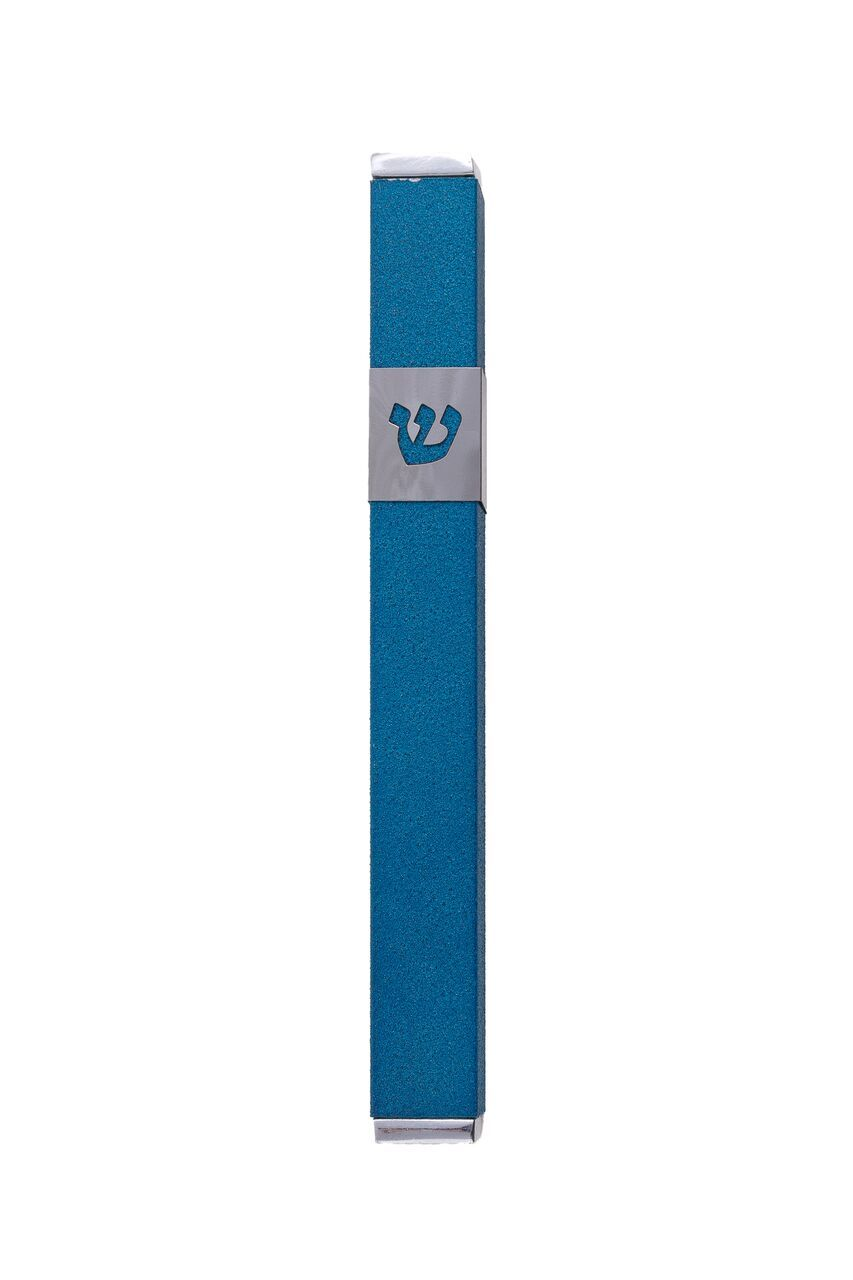one reason why it appears on all Mezuzot, often instead of the full Name.
The Arizal often equated Elohim(אלהים) with 300 because 300 is its value when the 5 letters are spelled-out.
Is it just hyper-efficiency that utilizes every letter and quantitative number in so many telling and revealing ways? Or are we just seeing glimpses of complex schematic diagrams of technology that we do yet comprehend?
Regardless, the 304,805 letters in the Torah represent 78.0% of the 390,625 words, letters, and verses in the Torah. Conversely, this means that the words and verses of the Torah represent 22% of those 390,625 elements, apropos considering there are 22 letters in the alphabet.
We have already seen some mathematical constants and some complicated calculations linked to the Torah, enough to prove it was created by an advanced intelligence and not our ancestors. So we know that that intelligence did not originate on Earth.  We could take the Torah then at face value, which says that G-d Created it. Regardless, before we go inward into the depths of the Torah, we can at least assume that whoever did create the Torah also at a minimum knew about the physical modeling of our universe.  If so, might He also build some of those modeling ratios and/or dimensions into our fixed Torah numbers? This would further demonstrate proof of intelligent design.
As it turns out, squaring the 304,805 letters of the Torah gives us the average distance from the Earth to the Sun, 92,955,807 miles, within 80 letters of accuracy. Given the Earth’s elliptical orbit around the Sun at 66,600 mph this is well within an acceptable range.  It is also remarkable that this nearly 93-million-mile distance is off by precisely 80 letters, the value (80) being that of the letter Pe (פ), which was highlighted by the 4,805 letter Pe’s (פ) of the total 304,805 letters.  It is almost as if the universe was proving its own point.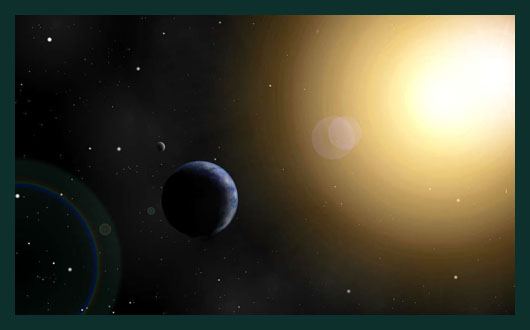That great distance is measured in terms of the speed of light as it travels from the Sun to the Earth called an Astronomical Unit(AU) and we have made all distances in our solar systems a multiple of it. Given that 173 is the small gematria of the 42-Letter Name, how odd is it that Man calculates the speed of light as 173.1446326847 AU/day and the distance from the Sun to the Earth is 1 AU.  And let us not forget that nine (9) divided by the same 42-Letter Name spelled out is 9/.1230490… = 73.141593, quite similar to 173.1446326.
This distance in miles works out to a standard 149597870700meters, a possible reference to the sum of the 22 letters, 1495, while in miles the speed of light is 670,616,629.424 mph…., reflecting the 670 paragraphs of “The Torah” of numerical value 616 and 424, Moshiach Ben David.
Beyond our solar system Man measures distance in light-years,the distance light travels in one year. The distance from Earth to the nearest star is 4.2 light years away. Larger distances are measured in parsecs of 3.26 light-years each, which makes that same distance to the nearest star, proxima centauri 4.244 parsecs. Perhaps, once we better understand the schematics, we will better understand the universe we are in.Our options of understanding are the following: 1) Ignore these relationships and assume they are just coincidental; 2) Figure that whoever created the Torah knew the dimensions of our solar system and the speed of light, etc, and moreover that He knew that Man would eventually after thousands of years adopt the specific time keeping and measuring systems that we actually did, in other words that He either knew our future or seeded it along the way; and option 3) Assume that whoever Created the Torah created everything.

## Partitioning the Letters

Let us get back to earth and dig deeper into the Torah. In general, in this paper, we are talking about sets or groupings and different ways to partition (split them up) them. We could group and partition any of the Torah elements and get absolutely fascinating results that defy logic, unless logic tells us they are adhering to some model of purposeful design, perhaps a circuitry of sorts, or a network. For example, each letter in the Torah is found a specific and defined number of times, like the set of all Pe’s (פ) in the Torah, and when we partitioned them into two sets, we got one set of 300,000 letters and the other with the 4,805 letter Pe’s (פ).  Of the remaining 21 Letters, exactly 90,100 of them comprise the set of Yuds (י), Heis (ה), and Vavs (ו), the component blocks of the Tetragrammaton (יהוה). These 3 important letters comprise 30.03330% of the total set of 300,000 letters, which is oddly reminiscent of the 3003 gematria of the Torah’s first 33 letters, which equaled the sum of the integers from 1 to 77. What is more astounding about that set of 90,100 letters is that it is the sum of the integers from 1 – 424, the numerical value of Moshiach Ben David.
Speaking of 3003, if you recall, when we first compared our original odd-even alef-bet split to the phi one, we saw that they were off by a pretty round number, which had hinted at the use of phi in the first place. Knowing what we now know, it makes sense that collectively the two splits were off from each other by .3003

```Odd/Even Splits
625/1495 was .4180
625/870 was   .7183
They are off from Phi (.6180) by:
.6180 - .4180 = .2000
.7183 - .6180 = .1003
.3003```

Each time we divide the remaining letters into new groupings we are separating them into subsets. We will break that subset of 90,100 letters down into further subsets, but first let us examine the relationship between this tiny subset of 33 letters and the completely separate one of 90,100.  Somehow, dividing the sum of the digits from 1 – 424 by the sum from 1- 77 yields 30.00333000.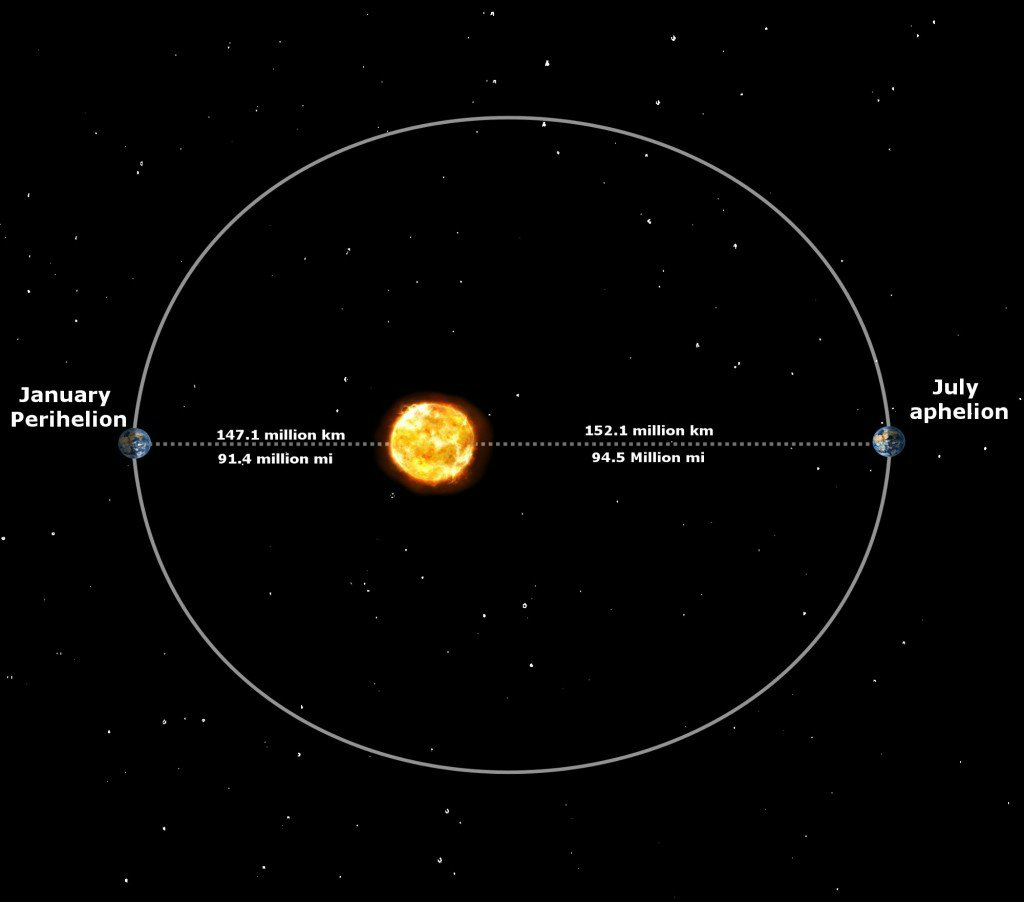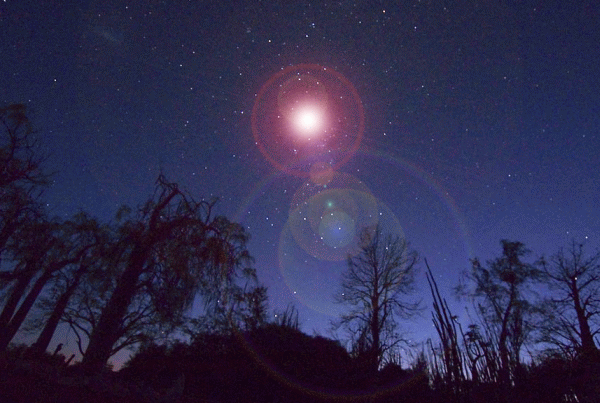If we want to bring prime numbers into this, or better said, if we want to see how prime numbers were woven into this equation since the Torah’s inception, we have to look no further than the face that the Torah’s first 33 letters, which equaled the sum of the integers from 1 to 77 are also the Torah’s first 8 words. The sum of the first 8 Primes (2 + 3 + 5 + 7 +11 +13 + 17 + 19) = 77.
Then when we divide the two resultant values by each other, 77/424, we get .18160377358… There is much to parse in this long fractional number, though 181 is relevant enough: as 181 is the 42nd Prime Number; and 181 is also the sum of the first 42 digits in the set of Prime Numbers; and as we will soon see the 42-Letter Name is directly related to those 90,100 Yuds (י), Heis (ה), and Vavs (ו) that gave us the number 424.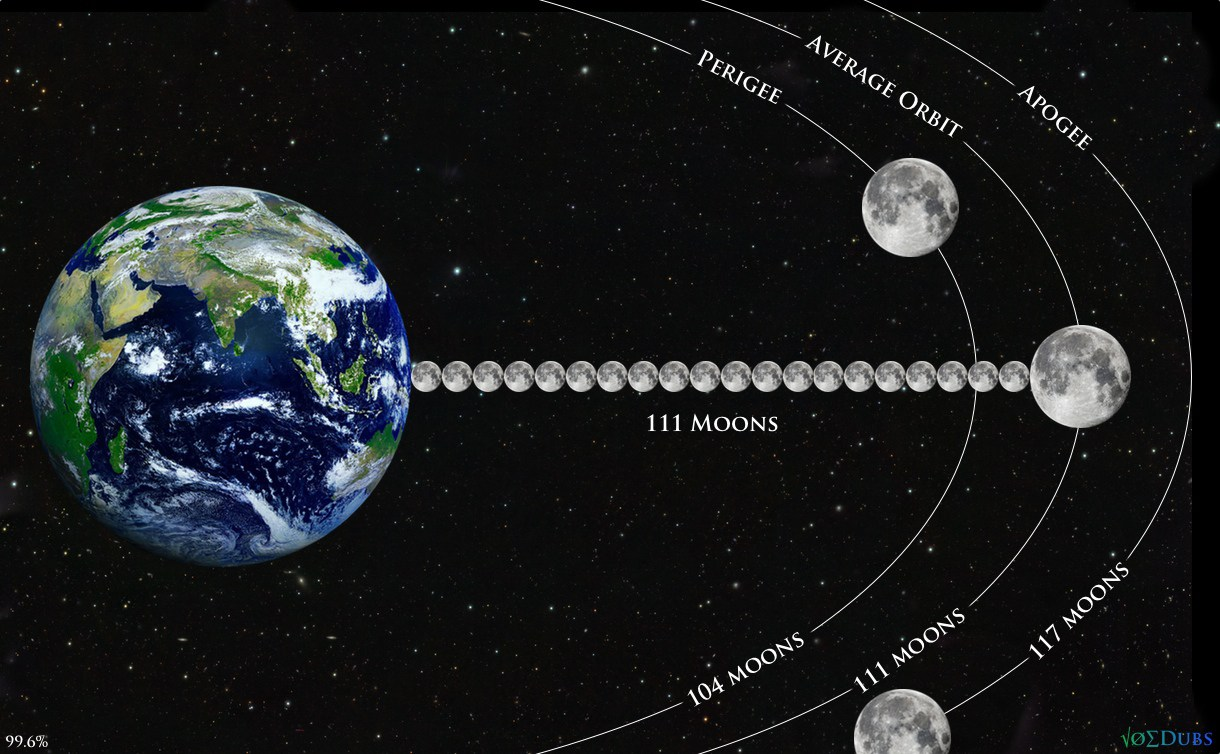Moreover, while the sum of the digits through 181 (the 42nd prime) is 378, and 378 is the gematria of the famous palindromic phrase “He and His Name are One (Echad Usho Echad)”, which we will also discuss shortly, when we add the 42 to 378, we get 378 +42 = 420.  This seems all the more relevant since there are 181 digits in 111! (factorial) and 111 is the numerical value of alef(אלפ), One(1), where it all begins.
Is there any specific reason why the Earth is exactly 111 Moon diameters from the Sun in its average orbit?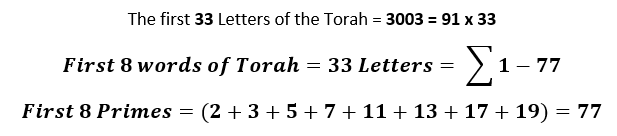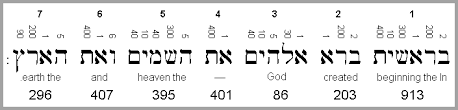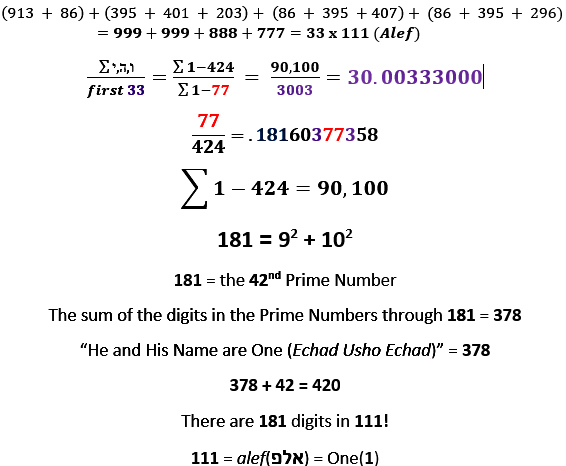Then again, if we were to add the relevant number 42 to 181, we would get 223, which is the difference between the 603773 in the result .181603773.. and the census taken in in Bamidbar 1:1 – 4:20 that gave us 603550 men counted.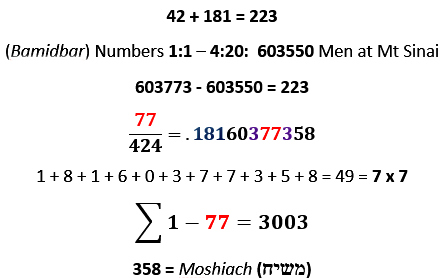This last point will become much more significant later in this paper, though for now, 181 is also the sum of two consecutive squares in that 181 = 92 + 102, reflective to be sure of the 90,100-letter subset we started with. What is being shown to us is that there is strong relationship between the numbers 77 and 424 and this somehow ties into the concept of an Anointed One. What we are about to see is how the Name of G-d (יהוה) also ties in.So now let us divide that important 90,100-letter subset of Yuds (י), Heis (ה), and Vavs (ו) into the 7280 Yuds (י), Heis (ה), and Vavs (ו) that we utilized to construct the actual 1820 Tetragrammatons (יהוה) in the Torah.  Before we go any further, we need to note that the numerical value of each Tetragrammaton (יהוה) is 26 and that the higher Name Ehyeh(אהיה) is found only 7 times in the Torah, yet when we divide 1820 by 7 we get exactly 260 or 26 x 10. There are exactly 260 Tetragrammaton (יהוה) for each Name Ehyeh(אהיה).  Moreover, when we divide the numerical value of the Tetragrammaton (יהוה) by the numerical value of the Name Ehyeh(אהיה) or 26/21, we get 1.238095…, which should set off alarm bells. This was the same 1/42 remainder we saw with 13/42 and with the two partitioning equations of the 22 letters into the 3 Mothers, 7 Doubles and 12 Elemental letters.  Only this time, this derivative of 42 is paired with the number 1.
Stop right here. The 3 most powerful Names of G-d are integrated into a single super simple equation. Yes, it breaks down to simple arithmetic but not if you use the Names rather than the numerical values that represent them: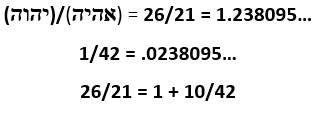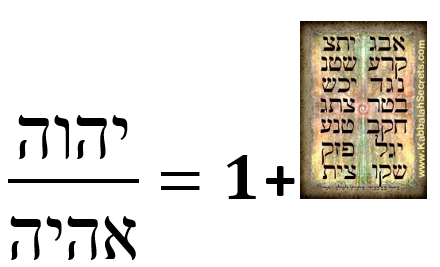Back to the 7280 Yuds (י), Heis (ה), and Vavs (ו). That the number 7280 came up again as we saw with the 72 Triplet square roots and with the 7-word 28-letter first verse of the Torah is significant, as is the sum of 7280 + 1820 = 9100, yet again a reflection of our 90,100-letter subset, keeping in mind these latest subsets are describing nothing less than the ineffable Name of G-d (יהוה). When we listen to the Torah and the reader gets to the unpronounceable Name (יהוה) he says “Adonai” and there are exactly 91 Adonai’s (אדני) in the Torah, matching the sum of the numerical value of Tetragrammaton (יהוה) and Adonai (אדני) together (26 + 65 = 91).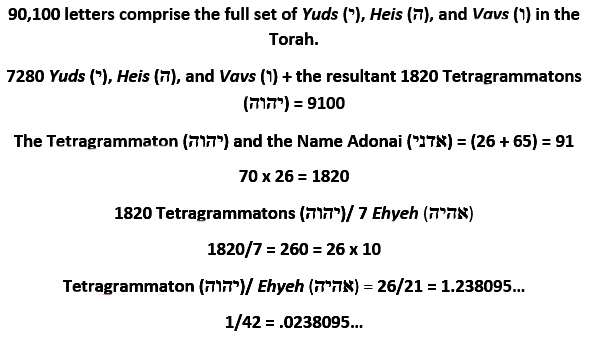For much more and much deeper work, including how the first word in the Torah, when spelled out also equals 1820 on this subject see Choose Redemption Now.
By the way, those 187 chapters, 670 paragraphs and 54 portions add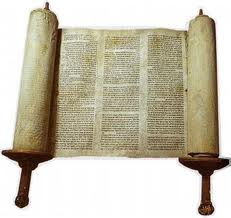up to 911. There may be another way to dial for emergency help beside your phone. Interestingly, when we divide the three(3) main written elements of the Torah by the three(3) main formal partitioning elements of the Torah in order of size, we get : the number of verses 5845 divided by the 54 portions; and the 79975 words in the Torah divided by the 187 Chapters; and the 304805 letters divided by the 670 paragraphs. The result of these three divisions (5845/54 + 79975/187 + 304805/670) and add (187 + 670 + 54) divided by 102 is 999.96 or 1000 yet again.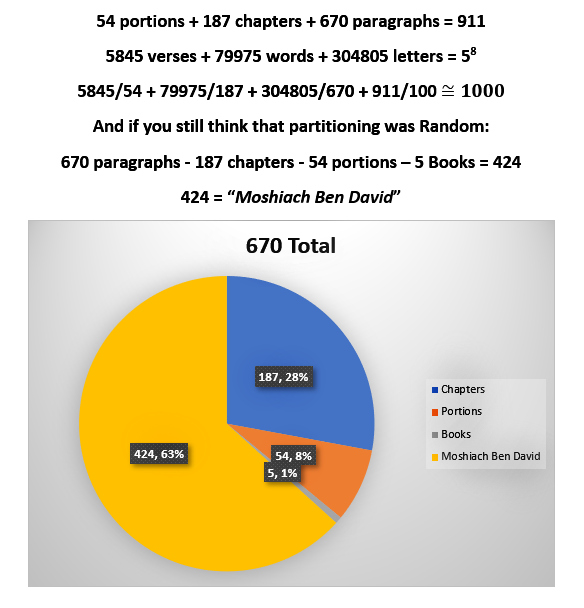The numbers themselves convey information to us, but it is the relationship between them that is really important. Your parents are important as individuals, but to you it is the fact that they are your parents that really matters, your relationship to them. In mathematics, relationship are called equations. As such, we see that there is a binding relationship between the 670 paragraphs, 187 chapters and the 54 portions that leads to Moshiach: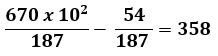We can also note that 670 – 54 = 616, the numerical value of “The Torah.”
A much more interesting and revealing equation is found in the simple relationship between the 248 columns and the 670 paragraphs: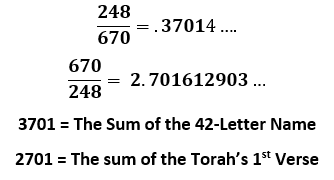The 42 Letters of the 42-Letter name add up to 3701 while the 28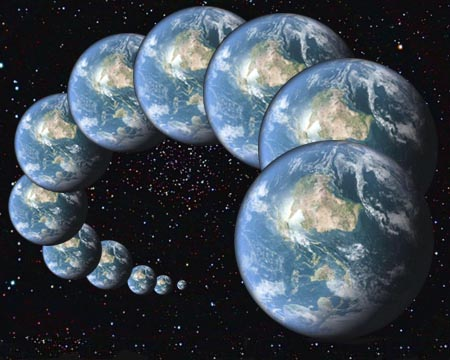Letters of the Torah’s first verse add up to 2701.  Now that a significant relationship has been established, we can use gematria to connect a few more dots. For example, in 2701612903, the triplet 612 is the gematria value for the word brit (ברית), meaning covenant, and 903 is the sum of all the integers from 1 – 42.  Since we know the 42-Letters of the Name are derived from the first 42 letters of the Torah we can see how one message embedded in this relationship is that the Covenant of 42 is found in the first verse. Moreover, now we can understand the relationship between the 1000 letters of the Shema and the 248 words of the Shema since 3701 – 2701 = 1000.

Of course, there are all sorts of ways of partitioning the Torah. We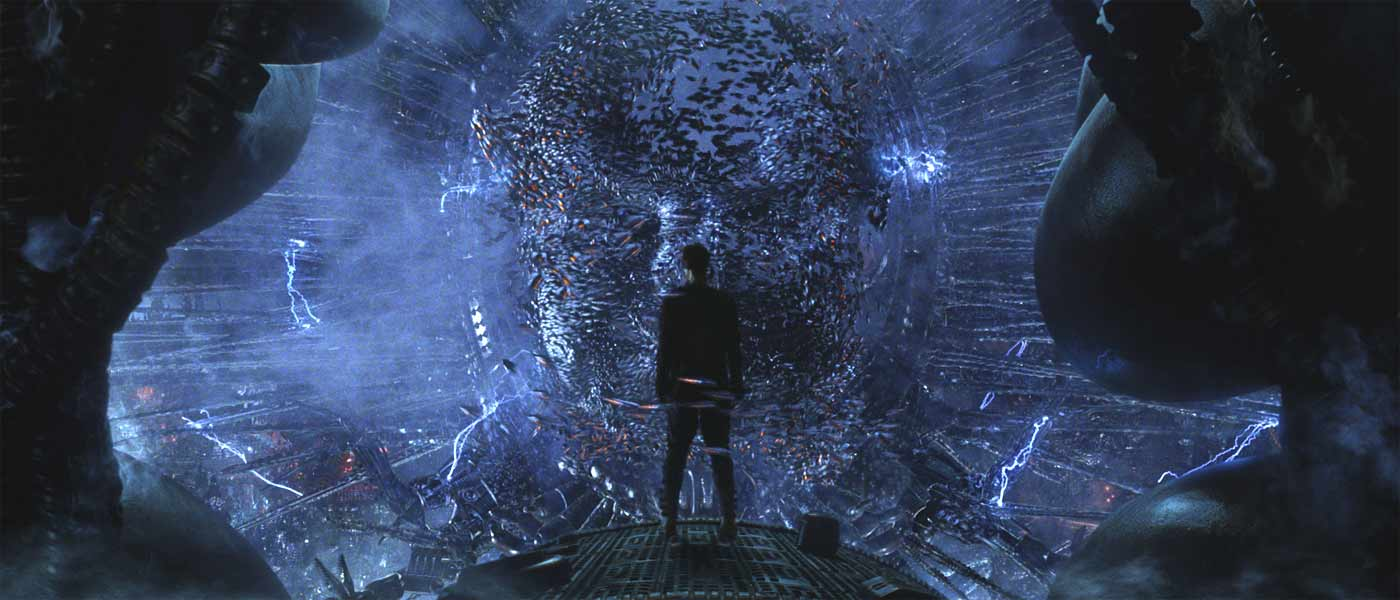have only to look and we will find them. There is a saying in Kabbalah that when the student is ready the teacher will come. This refers to the personal training and connection one must make and is why it was has always been taught with a few notable exceptions one-on-one and not through mass marketing. We should make the disclaimer here that we are not teaching Kabbalah in these articles, we are revealing the Torah and the universe in a light that penetrates the darkness of the illusions. We use transparently use certain kabbalistic tools to help make those revelations and in so doing help others to use them to. The teacher-student relationship is the one you can make with the Torah, learning and downloading deep ancient knowledge directly one-on-one, passed down directly not through new age aphorisms.That said, let us peer into the Torah and get a glimpse of what it can teach us through yet another partitioning.  If we partition those 187 chapters into its first 3 and the remaining 184, which is also the same as separating the 670 paragraphs into the first 11 paragraphs and remaining 659, we find that they end precisely at the pivotal moment with Man being kicked out of the Garden of Eden. Up to this this natural and momentous breaking point in the Genesis narrative there are exactly 4213 letters. It may be a message to us of what we will need to survive, 42 and 13, or what we need to get back in?